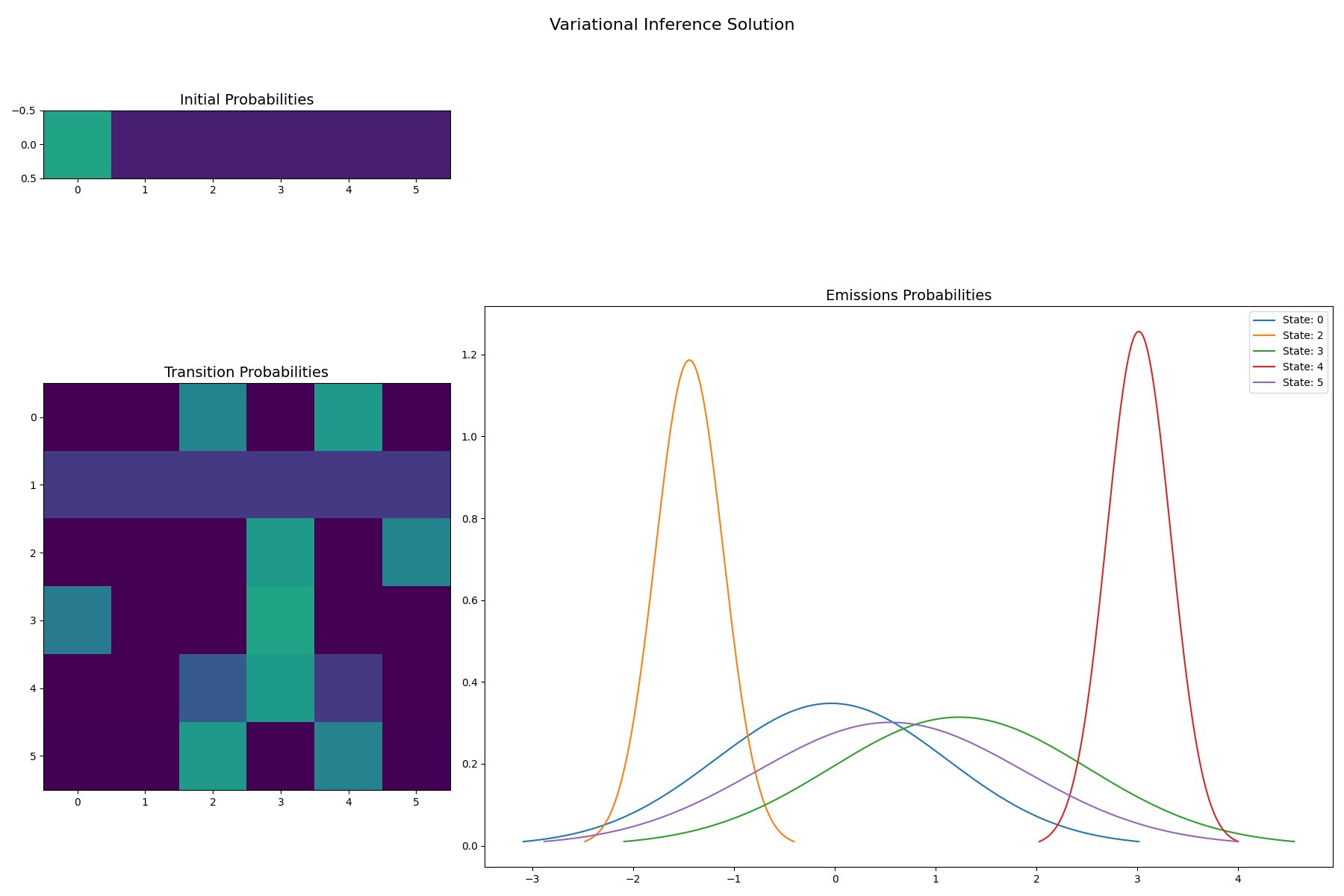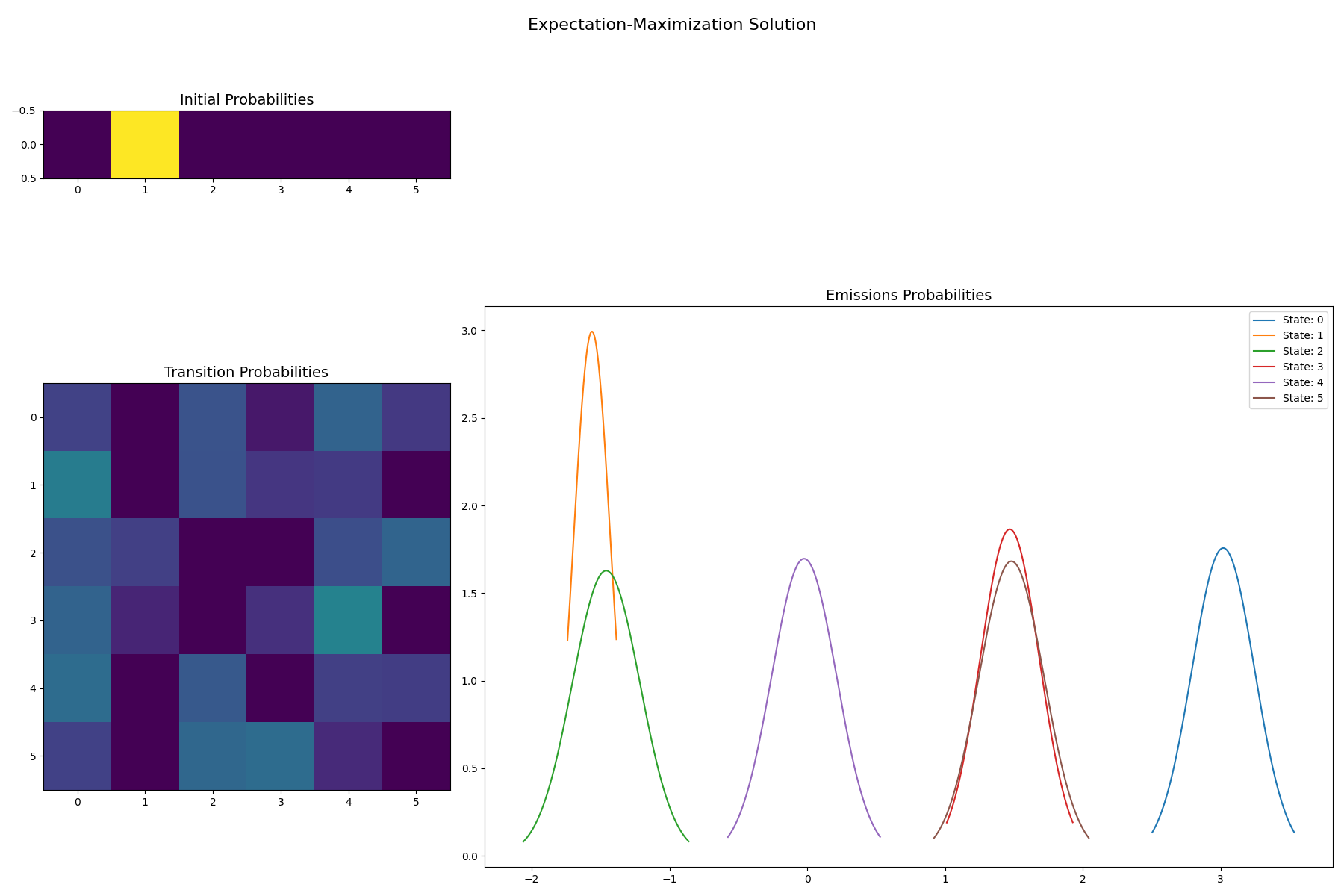# Learning an HMM using VI and EM over a set of Gaussian sequences#

We train models with a variety of number of states (N) for each algorithm, and then examine which model is “best”, by printing the log-likelihood or variational lower bound for each N. We will see that an HMM trained using VI will prefer the correct number of states, while an HMM learning with EM will prefer as many states as possible. Note, for models trained with EM, some other criteria such as AIC/BIC, or held out test data, could be used to select the correct number of hidden states.

••```Training VI(1) Variational Lower Bound=-528.5243461575363 Iterations=3
Training EM(1) Final Log Likelihood=-978.1330363616133 Iterations=3
Training VI(1) Variational Lower Bound=-528.5243461575363 Iterations=3
Training EM(1) Final Log Likelihood=-978.1330363616133 Iterations=3
Training VI(1) Variational Lower Bound=-528.5243461575363 Iterations=3
Training EM(1) Final Log Likelihood=-978.1330363616133 Iterations=3
Training VI(1) Variational Lower Bound=-528.5243461575363 Iterations=3
Training EM(1) Final Log Likelihood=-978.1330363616133 Iterations=3
Training VI(1) Variational Lower Bound=-528.5243461575363 Iterations=3
Training EM(1) Final Log Likelihood=-978.1330363616133 Iterations=3
Training VI(2) Variational Lower Bound=-466.26523164915716 Iterations=21
Training EM(2) Final Log Likelihood=-918.9148218161756 Iterations=21
Training VI(2) Variational Lower Bound=-486.09498490626356 Iterations=53
Model is not converging.  Current: -882.4310081762973 is not greater than -882.4309848701383. Delta is -2.3306158936975407e-05
Training EM(2) Final Log Likelihood=-882.4310081762973 Iterations=53
Training VI(2) Variational Lower Bound=-486.0949849880572 Iterations=54
Training EM(2) Final Log Likelihood=-918.9148216250994 Iterations=54
Training VI(2) Variational Lower Bound=-484.3987460065557 Iterations=21
Training EM(2) Final Log Likelihood=-918.91482197061 Iterations=21
Training VI(2) Variational Lower Bound=-486.09498546349005 Iterations=42
Training EM(2) Final Log Likelihood=-863.4014824857761 Iterations=42
Training VI(3) Variational Lower Bound=-402.1045219981162 Iterations=115
Training EM(3) Final Log Likelihood=-776.3769231275905 Iterations=115
Training VI(3) Variational Lower Bound=-433.6611898993304 Iterations=47
Model is not converging.  Current: -776.3768325595769 is not greater than -776.3768212571706. Delta is -1.1302406278446142e-05
Training EM(3) Final Log Likelihood=-776.3768325595769 Iterations=47
Training VI(3) Variational Lower Bound=-491.36604338559533 Iterations=73
Training EM(3) Final Log Likelihood=-917.2393418090232 Iterations=73
Training VI(3) Variational Lower Bound=-489.410770086351 Iterations=30
Training EM(3) Final Log Likelihood=-802.192945794781 Iterations=30
Training VI(3) Variational Lower Bound=-471.3061935219601 Iterations=22
Training EM(3) Final Log Likelihood=-779.6200733661288 Iterations=22
Training VI(4) Variational Lower Bound=-520.6487316255037 Iterations=65
Training EM(4) Final Log Likelihood=-657.698512985182 Iterations=65
Training VI(4) Variational Lower Bound=-421.0695755064629 Iterations=171
Model is not converging.  Current: -657.6985124018764 is not greater than -657.6985100704595. Delta is -2.3314169084187597e-06
Training EM(4) Final Log Likelihood=-657.6985124018764 Iterations=171
Training VI(4) Variational Lower Bound=-438.3707550155327 Iterations=72
Training EM(4) Final Log Likelihood=-769.7550887718468 Iterations=72
Training VI(4) Variational Lower Bound=-494.47047868440666 Iterations=50
Training EM(4) Final Log Likelihood=-657.6985130041445 Iterations=50
Training VI(4) Variational Lower Bound=-503.6272469277964 Iterations=160
Training EM(4) Final Log Likelihood=-774.1825251051414 Iterations=160
Training VI(5) Variational Lower Bound=-458.2637326447116 Iterations=41
Training EM(5) Final Log Likelihood=-652.5634291453131 Iterations=41
Training VI(5) Variational Lower Bound=-474.3337709493111 Iterations=42
Training EM(5) Final Log Likelihood=-649.898840267143 Iterations=42
Training VI(5) Variational Lower Bound=-481.587385853616 Iterations=25
Training EM(5) Final Log Likelihood=-648.0188620315693 Iterations=25
Training VI(5) Variational Lower Bound=-521.5066678665968 Iterations=142
Training EM(5) Final Log Likelihood=-651.6797940221901 Iterations=142
Training VI(5) Variational Lower Bound=-517.4780929140921 Iterations=61
Training EM(5) Final Log Likelihood=-652.5634257449441 Iterations=61
Training VI(6) Variational Lower Bound=-500.6856594755132 Iterations=106
Training EM(6) Final Log Likelihood=-645.1814332463392 Iterations=106
Training VI(6) Variational Lower Bound=-508.40925927173805 Iterations=46
Model is not converging.  Current: -640.0165379600729 is not greater than -640.0165340760798. Delta is -3.88399314488197e-06
Training EM(6) Final Log Likelihood=-640.0165379600729 Iterations=46
Training VI(6) Variational Lower Bound=-542.5468927811029 Iterations=14
Training EM(6) Final Log Likelihood=-638.3316230616362 Iterations=14
Training VI(6) Variational Lower Bound=-531.8924113271744 Iterations=45
Training EM(6) Final Log Likelihood=-638.3167166182112 Iterations=45
Training VI(6) Variational Lower Bound=-516.7147487691999 Iterations=91
Training EM(6) Final Log Likelihood=-638.3167166433591 Iterations=91
VI(1): -528.5243
VI(2): -466.2652
VI(3): -402.1045* <- Best Model
VI(4): -421.0696
VI(5): -458.2637
VI(6): -500.6857
Best Model VI
[[0.261 0.505 0.235]
[0.225 0.455 0.319]
[0.218 0.584 0.198]]
[[1.482]
[-0.708]
[3.001]]
[[[0.051]]

[[0.663]]

[[0.090]]]
EM(1): -978.1330
EM(2): -863.4015
EM(3): -776.3768
EM(4): -657.6985
EM(5): -648.0189
EM(6): -638.3167* <- Best Model
Best Model EM
[[0.197 0.258 0.227 0.202 0.000 0.116]
[0.282 0.202 0.271 0.000 0.020 0.226]
[0.238 0.305 0.125 0.129 0.204 0.000]
[0.378 0.000 0.305 0.000 0.000 0.317]
[0.245 0.000 0.026 0.000 0.000 0.729]
[0.337 0.455 0.101 0.107 0.000 0.000]]
[[3.020]
[-1.480]
[1.468]
[-0.006]
[1.520]
[-0.034]]
[[[0.051]]

[[0.053]]

[[0.050]]

[[0.047]]

[[0.063]]

[[0.061]]]
VI solution for 6 states: Notice sparsity among states 1 and 4
[[0.007 0.007 0.007 0.965 0.007 0.007]
[0.006 0.006 0.006 0.969 0.006 0.006]
[0.001 0.001 0.180 0.656 0.001 0.159]
[0.001 0.001 0.314 0.397 0.114 0.174]
[0.006 0.006 0.006 0.671 0.006 0.305]
[0.346 0.393 0.253 0.002 0.002 0.003]]
[[0.026]
[1.777]
[-1.467]
[1.292]
[-0.045]
[2.993]]
[[[0.073]]

[[0.704]]

[[0.097]]

[[1.569]]

[[0.067]]

[[0.112]]]
EM solution for 6 states
[[0.197 0.258 0.227 0.202 0.000 0.116]
[0.282 0.202 0.271 0.000 0.020 0.226]
[0.238 0.305 0.125 0.129 0.204 0.000]
[0.378 0.000 0.305 0.000 0.000 0.317]
[0.245 0.000 0.026 0.000 0.000 0.729]
[0.337 0.455 0.101 0.107 0.000 0.000]]
[[3.020]
[-1.480]
[1.468]
[-0.006]
[1.520]
[-0.034]]
[[[0.051]]

[[0.053]]

[[0.050]]

[[0.047]]

[[0.063]]

[[0.061]]]
```

```import collections
import numpy as np
import matplotlib.pyplot as plt
import matplotlib.gridspec as gs
import scipy.stats

from sklearn.utils import check_random_state

from hmmlearn import hmm, vhmm
import matplotlib

def gaussian_hinton_diagram(startprob, transmat, means,
variances, vmin=0, vmax=1, infer_hidden=True):
"""
Show the initial state probabilities, the transition probabilities
as heatmaps, and draw the emission distributions.
"""

num_states = transmat.shape

f = plt.figure(figsize=(3*(num_states), 2*num_states))
grid = gs.GridSpec(3, 3)

ax.imshow(startprob[None, :], vmin=vmin, vmax=vmax)
ax.set_title("Initial Probabilities", size=14)

ax.imshow(transmat, vmin=vmin, vmax=vmax)
ax.set_title("Transition Probabilities", size=14)

for i in range(num_states):
keep = True
if infer_hidden:
if np.all(np.abs(transmat[i] - transmat[i]) < 1e-4):
keep = False
if keep:
s_min = means[i] - 10 * variances[i]
s_max = means[i] + 10 * variances[i]
xx = np.arange(s_min, s_max, (s_max - s_min) / 1000)
norm = scipy.stats.norm(means[i], np.sqrt(variances[i]))
yy = norm.pdf(xx)
keep = yy > .01
ax.plot(xx[keep], yy[keep], label="State: {}".format(i))
ax.set_title("Emissions Probabilities", size=14)
ax.legend(loc="best")
f.tight_layout()
return f

np.set_printoptions(formatter={'float_kind': "{:.3f}".format})
rs = check_random_state(2022)
sample_length = 500
num_samples = 1
# With random initialization, it takes a few tries to find the
# best solution
num_inits = 5
num_states = np.arange(1, 7)
verbose = False

# Prepare parameters for a 4-components HMM
# And Sample several sequences from this model
model = hmm.GaussianHMM(4, init_params="")
model.n_features = 4
# Initial population probability
model.startprob_ = np.array([0.25, 0.25, 0.25, 0.25])
# The transition matrix, note that there are no transitions possible
# between component 1 and 3
model.transmat_ = np.array([[0.2, 0.2, 0.3, 0.3],
[0.3, 0.2, 0.2, 0.3],
[0.2, 0.3, 0.3, 0.2],
[0.3, 0.3, 0.2, 0.2]])
# The means and covariance of each component
model.means_ = np.array([[-1.5],
,
[1.5],
[3.]])
model.covars_ = np.array([[0.25],
[0.25],
[0.25],
[0.25]])**2

# Generate training data
sequences = []
lengths = []

for i in range(num_samples):
sequences.extend(model.sample(sample_length, random_state=rs))
lengths.append(sample_length)
sequences = np.asarray(sequences)

# Train a suite of models, and keep track of the best model for each
# number of states, and algorithm
best_scores = collections.defaultdict(dict)
best_models = collections.defaultdict(dict)
for n in num_states:
for i in range(num_inits):
vi = vhmm.VariationalGaussianHMM(n,
n_iter=1000,
covariance_type="full",
implementation="scaling",
tol=1e-6,
random_state=rs,
verbose=verbose)
vi.fit(sequences, lengths)
lb = vi.monitor_.history[-1]
print(f"Training VI({n}) Variational Lower Bound={lb} "
f"Iterations={len(vi.monitor_.history)} ")
if best_models["VI"].get(n) is None or best_scores["VI"][n] < lb:
best_models["VI"][n] = vi
best_scores["VI"][n] = lb

em = hmm.GaussianHMM(n,
n_iter=1000,
covariance_type="full",
implementation="scaling",
tol=1e-6,
random_state=rs,
verbose=verbose)
em.fit(sequences, lengths)
ll = em.monitor_.history[-1]
print(f"Training EM({n}) Final Log Likelihood={ll} "
f"Iterations={len(vi.monitor_.history)} ")
if best_models["EM"].get(n) is None or best_scores["EM"][n] < ll:
best_models["EM"][n] = em
best_scores["EM"][n] = ll

# Display the model likelihood/variational lower bound for each N
# and show the best learned model
for algo, scores in best_scores.items():
best = max(scores.values())
best_n, best_score = max(scores.items(), key=lambda x: x)
for n, score in scores.items():
flag = "* <- Best Model" if score == best_score else ""
print(f"{algo}({n}): {score:.4f}{flag}")

print(f"Best Model {algo}")
best_model = best_models[algo][best_n]
print(best_model.transmat_)
print(best_model.means_)
print(best_model.covars_)

# Also inpsect the VI model with 6 states, to see how it has sparse structure
vi_model = best_models["VI"]
em_model = best_models["EM"]
print("VI solution for 6 states: Notice sparsity among states 1 and 4")
print(vi_model.transmat_)
print(vi_model.means_)
print(vi_model.covars_)
print("EM solution for 6 states")
print(em_model.transmat_)
print(em_model.means_)
print(em_model.covars_)

f = gaussian_hinton_diagram(
vi_model.startprob_,
vi_model.transmat_,
vi_model.means_.ravel(),
vi_model.covars_.ravel(),
)
f.suptitle("Variational Inference Solution", size=16)
f = gaussian_hinton_diagram(
em_model.startprob_,
em_model.transmat_,
em_model.means_.ravel(),
em_model.covars_.ravel(),
)
f.suptitle("Expectation-Maximization Solution", size=16)

plt.show()
```

Total running time of the script: ( 0 minutes 18.231 seconds)

Gallery generated by Sphinx-Gallery